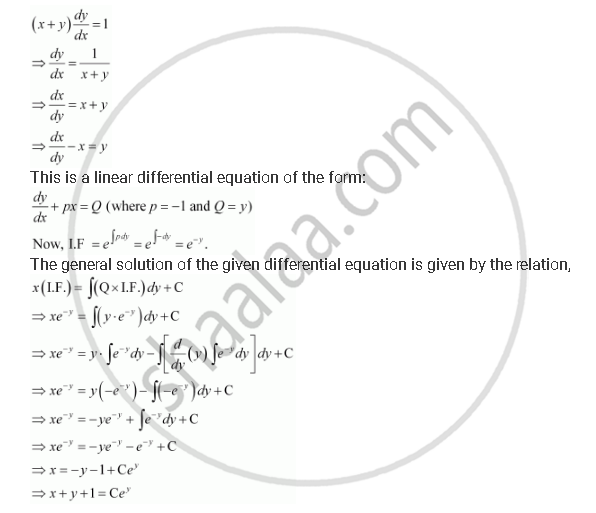Share

# For the Differential Equations Find the General Solution: (X + Y) Dy/Dx = 1 - CBSE (Commerce) Class 12 - Mathematics

ConceptMethods of Solving First Order, First Degree Differential Equations Linear Differential Equations

#### Question

For the differential equations find the general solution:

(x + y) dy/dx = 1

#### SolutionIs there an error in this question or solution?

#### APPEARS IN

NCERT Solution for Mathematics Textbook for Class 12 (2018 to Current)
Chapter 9: Differential Equations
Q: 10 | Page no. 414

#### Video TutorialsVIEW ALL 

Solution For the Differential Equations Find the General Solution: (X + Y) Dy/Dx = 1 Concept: Methods of Solving First Order, First Degree Differential Equations - Linear Differential Equations.
S